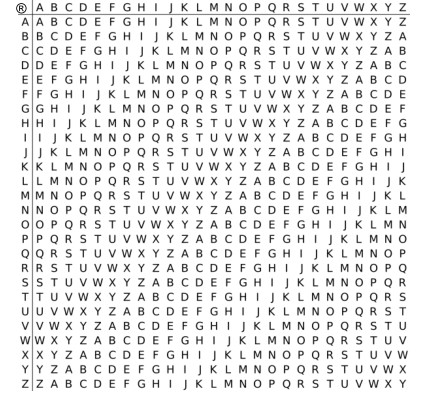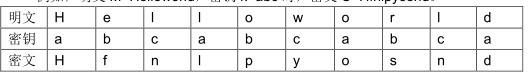# P1079 Vigenère 密码

• 7.9K通过
• 14.7K提交
• 题目提供者 CCF_NOI
• 评测方式 云端评测
• 标签 字符串 模拟 NOIp提高组 2012
• 难度 普及-
• 时空限制 1000ms / 128MB
• 提示：收藏到任务计划后，可在首页查看。

## 题目描述

16 世纪法国外交家 $Blaise de Vigenère$ 设计了一种多表密码加密算法――$Vigenère$ 密码。$Vigenère$ 密码的加密解密算法简单易用，且破译难度比较高，曾在美国南北战争中为南军所广泛使用。

在密码学中，我们称需要加密的信息为明文，用 $M$表示；称加密后的信息为密文，用$C$表示；而密钥是一种参数，是将明文转换为密文或将密文转换为明文的算法中输入的数据，记为$k$。 在 $Vigenère$ 密码中，密钥$k$是一个字母串，$k=k_1,k_2,…,k_n$。当明文 $M=m_1,m_2,…,m_n$时，得到的密文 $C=c_1,c_2,…,c_n$，其中$c_i$=$m_i$®$k_i$，运算®的规则如下表所示：$Vigenère$ 加密在操作时需要注意：

1. ®运算忽略参与运算的字母的大小写，并保持字母在明文$M$ 中的大小写形式；

2. 当明文 $M$ 的长度大于密钥 $k$ 的长度时，将密钥 $k$重复使用。

例如，明文 $M=Helloworld$，密钥$k=abc$ 时，密文 $C=Hfnlpyosnd$。## 输入输出格式

输入格式：

共 2 行。

第一行为一个字符串，表示密钥$k$，长度不超过$100$，其中仅包含大小写字母。

第二行为一个字符串，表示经加密后的密文，长度不超过$1000$，其中仅包含大小写字母。

输出格式：

一个字符串，表示输入密钥和密文所对应的明文。

## 输入输出样例

输入样例#1： 复制
CompleteVictory
Yvqgpxaimmklongnzfwpvxmniytm 
输出样例#1： 复制
Wherethereisawillthereisaway

## 说明

【数据说明】

对于 100%的数据，输入的密钥的长度不超过 $100$，输入的密文的长度不超过$1000$，且都仅包含英文字母。

NOIP 2012 提高组 第一天 第一题

提示
标程仅供做题后或实在无思路时参考。
请自觉、自律地使用该功能并请对自己的学习负责。
如果发现恶意抄袭标程，将按照I类违反进行处理。